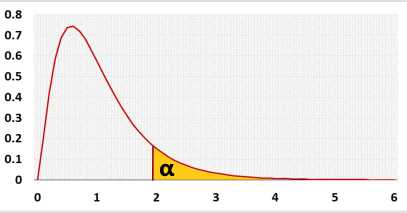Linear Regression Calculator

You may change the X and Y labels. Separate data by Enter or comma, , after each value. The tool ignores non-numeric cells.

Information

The linear regression calculator generates the linear regression equation, draws a linear regression line, a histogram, a residuals QQ-plot, a residuals x-plot, and a distribution chart.
It calculates the R square, the R, and the outliers, then it tests the fit of the linear model to the data and checks the residuals' normality assumption and the priori power.

What is linear regression?

The linear regression is the linear equation that best fits the points.
There is no one way to choose the best fit ting line, the most common one is the ordinary least squares (OLS). The linear regression describes the relationship between the dependent variable (Y) and the independent variables (X).
The linear regression model calculates the dependent variable (DV) based on the independent variables (IV, predictors).

What is "ordinary least squares"?

The ordinary least squares method chooses the line parameters that minimize the sum of squares of the differences between the observed dependent variables (Y) and the estimated value by the linear regression (Ŷ).

Why do you need the linear regression?

We may use the linear regression when we want to do one of the following
• Predict the dependent variable (Ŷ)
• Estimate the effect of each independent variable (X) on the dependent variable (Y)
• Calculate the correlation between the dependent variable and the independent variables.
• Test the linear model significance level.

How to calculate linear regression?

Following the linear regression formula:
Ŷ = b0 +b1x
b0 - the y-intercept, where the line crosses the y-axis.
b1 - the slope, describes the line's direction and incline.
 b1 = SPxy = Σ(xi-x̄)(yi-ȳ) SSx Σ(xi-x̄)2
b0 = ȳ - b1

How to calculate R squares?

R squares is the percentage of the variance explain by the regression (SSRegression) from the overall variance (SSTotal).
 R2 = SSRegression SSTotal

Linear regression in calculator

This online calculator supports all the basic functionality and more.

The right-tailed F test checks if the entire regression model is statistically significant. Why only right tail?
For Multiple regression calculator with the stepwise method and assumptions validations: multiple regression calculator

The following statistic checks if the linear regression model supports better results than the average of Y.
Hypotheses
H0: Y = b0
H1: Y = b0+b1X
Test statistic
 F = MS(regression) MS (residulas)
F distribution